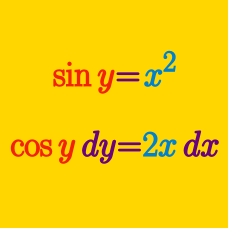Calculus

# Implicit Differentiation Problem Solving

Let $(a, b)$ be the intersection point of the curve $x^3+y^3+x^2y+7=0$ and the line $9x+y=0.$ What is the slope of the tangent line to the curve $x^3+y^3+x^2y+7=0$ at the point $(a, b)?$

If $\displaystyle y=\sqrt{\tan^{-1}(4x^{3})},$ what is $\displaystyle \frac{dy}{dx}?$

The equation of the line tangent to $(x^2 + y^2)^4 = 16x^2y^2$ at $(x,y) = (-1,1)$ is $ay = bx + c$, where $a$, $b$ and $c$ are positive integers. If $a = 1$, what is the value of $a + b + c$?

Given $\displaystyle e^{xy}=\sin (2x+7y),$ what is $\displaystyle \frac{dy}{dx}?$

Suppose $f(x)$ is a differentiable function that satisfies $f(1)=16.$ If the derivative of the function $g(x)=x \sqrt{f(x)}$ at the point $x=1$ is $5,$ what is the value of $f'(1)?$

×